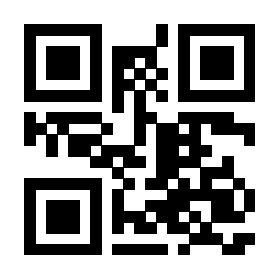# 5再提一个关于段落文本高亮显示的问题(利用xeCJKfntef宏包实现效果)

\documentclass[fontset=windows]{ctexart}
\usepackage{xeCJKfntef}
\usepackage{xcolor}
\newcommand{\hl}{\CJKunderanyline*{0.5ex}{\color{yellow}\rule{0.1pt}{2.5ex}}{#1}}
\begin{document}

$\mbox{\hl{y=f(x),x\in A.}}$
\end{document}$\hl{y=\int f(x),x\in A.}\quad f_{\hl{x}}$
\end{document}1 个回答

### 撰写答案